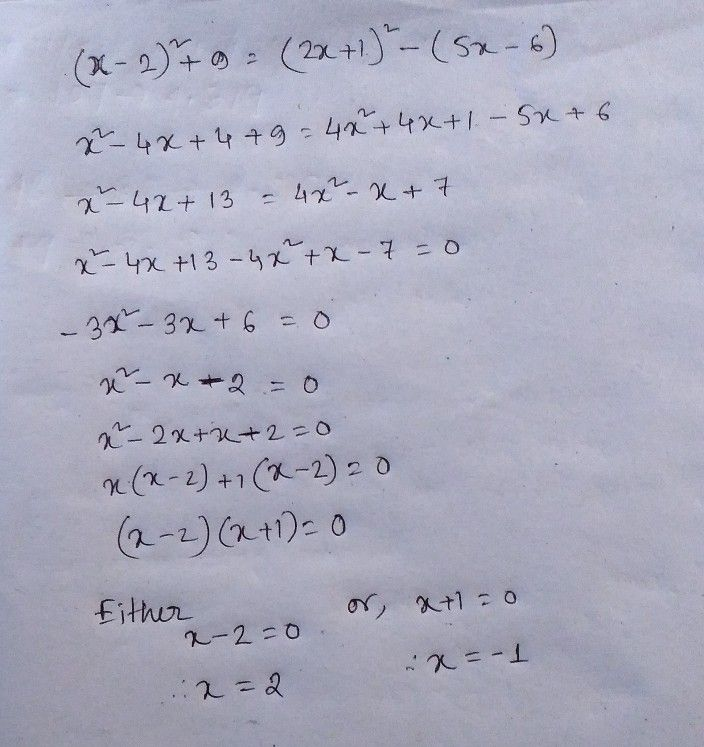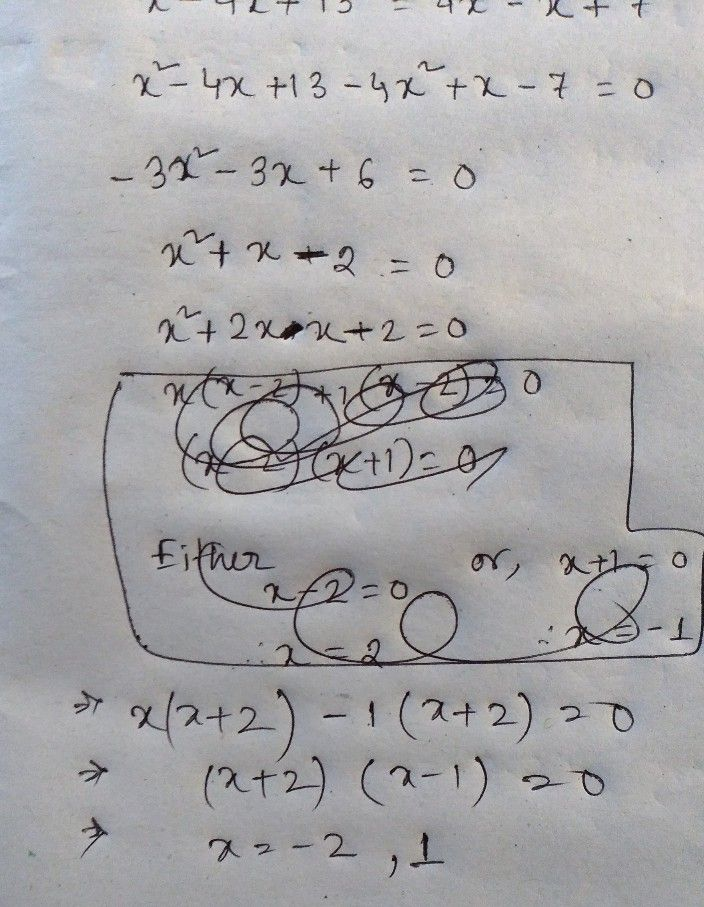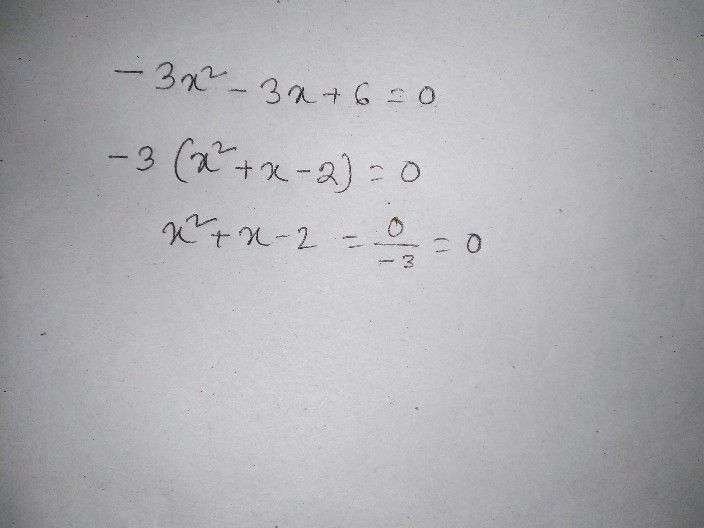Symbol
ProblemSolve $\left(a\right)\left(x-4\right)^{2}+5=\left(x+2\right)^{2}-\left(9-14x\right)$ $-1$ $\left(x-2\right)^{2}+9=\left(2x+1\right)^{2}-\left(5x-6\right)$ Solve $:\left(a\right)$ $\dfrac {2x-1} {5x+3}=\dfrac {2x+2} {5x+16}$ $0$ $\dfrac {4x+1} {3x+2}=\dfrac {4x+6} {3x+7}$ Solve $:\left(a\right)$ $\dfrac {x+2} {x+1}-\dfrac {x+2} {x-1}=\dfrac {x+5} {x^{2}-1}$ $\dfrac {2-x} {x+2}-\dfrac {2x-1} {x-2}=\dfrac {5x-1} {x^{2}-4}$
7th-9th grade
Algebra
Search count: 105
SolutionQanda teacher - LizaStudent
but in my -2 is there
bookQanda teacher - Liza
Let me checkyes there was an error dear ..sorry
i correct it
check
you understand?Student
haa thank sirQanda teacher - Liza
Please evaluate and give your valuable feedbackStudent
s sir but lastly somewhat I am not understandingQanda teacher - Liza
which part tell me specifically dear
i take common
in lastStudent
in 6 step 3 u didn't writeQanda teacher - Liza
x+2=0 hence x=-2
okay
i am doing againStudent
Kk sirQanda teacher - Lizayou understand nowStudent
then what is the final answer sirQanda teacher - Liza
x=-2 or 1Student
Kk sirQanda teacher - Liza
Okey then please evaluate
and if you have more questions then you can ask me separatelyStudent
kk
sir howQanda teacher - Liza
After evaluation you may ask me directly from preferred match sectionStudent
Kk then bye sirQanda teacher - Liza
OkeyStudent
Sir how get coins sir
I have no coins to give u then howQanda teacher - Liza
just give ratings here.Student
Kk s sir